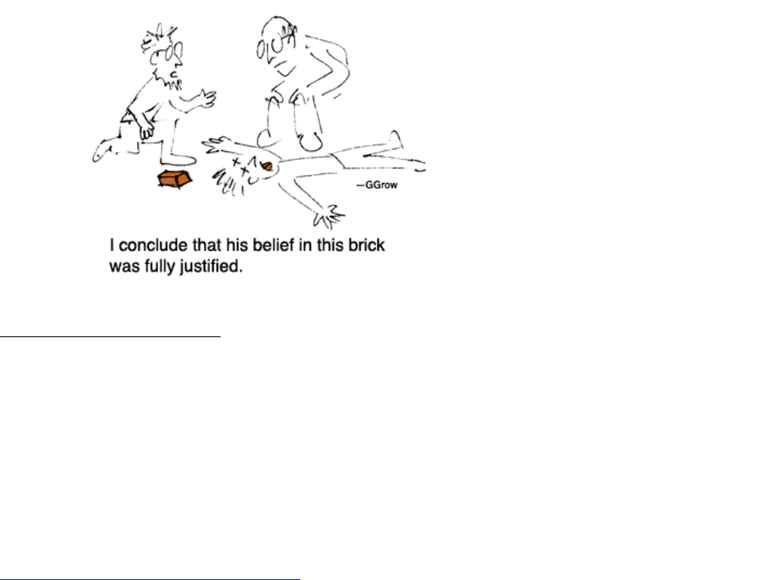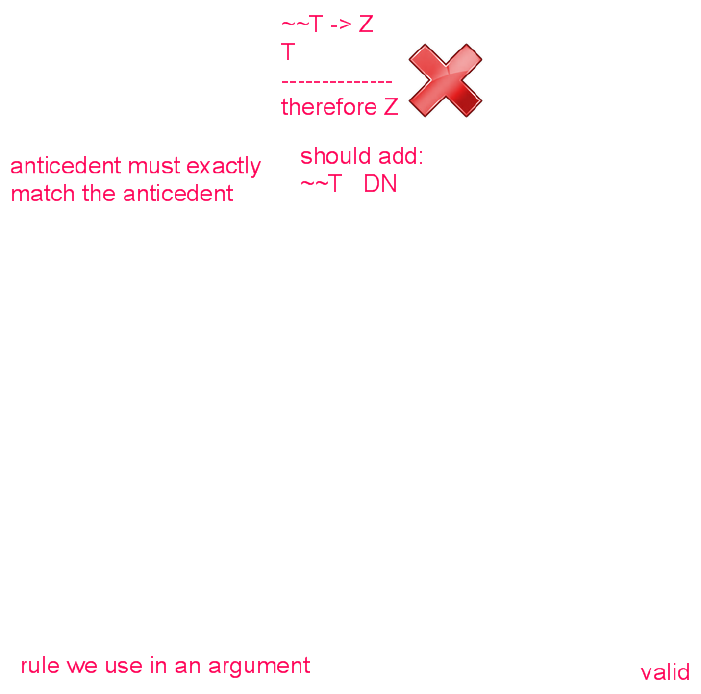Textbook Notes (280,000)
CA (170,000)
UTSG (10,000)
PHL (100)
Chapter

# Logic Unit 3 Part 1.pdf

Department
Philosophy
Course Code
PHL245H1
Professor
Niko Scharer

This preview shows pages 1-3. to view the full 39 pages of the document.Logic Unit 3 Part 1: Derivations with Negation and Conditional
2011 Niko Scharer
1
UNIT 3: DERIVATIONS FOR SENTENTIAL LOGIC
NATURAL DEDUCTION
Part 1
3.1 What is a derivation?
A derivation is a proof or demonstration that shows how a sentence or sentences can be derived
(obtained by making valid inferences) from a set of sentences. A derivation can be used to
demonstrate that an argument is valid; that a sentence is a tautology or that a set of sentences is
inconsistent. In a derivation, you are proving that the conclusion logically follows from the premises.
We’ll be using a natural deduction system for first-order logic that uses the symbolic language that we
have learned. Our system is based on that presented by Kalish and Montague in their text, Techniques
of Formal Reasoning.
1
All of our derivation rules are truth-preserving, so that if we follow the rules
and the premises are true, we can only derive true conclusions.
Every sentence that is logically entailed by a set of sentences can be derived from that set of sentences
using our derivation system the system is complete. Every one of the infinite number of valid
theorems and valid arguments (within the scope of first-order sentential logic) can be proven.
Every argument arrived at through our sentential derivation system will be deductively valid our
system is consistent. Thus, true premises will always lead to a true conclusion.
1
Kalish, Donald, and Montague, Richard, 1964. Logic: Techniques of Formal Reasoning. Harcourt, Brace, and
Jovanovich.
Our system is based on Kalish and Montague’s natural deduction system for first-order logic, an elegant logical
system that treats negation and material conditional as primary. It uses three types of derivation which we will
be learning in this unit: direct derivation, indirect derivation and conditional derivation. Except for a single rule
of inference (modus ponens) no other logical symbol or rule of inference is necessary to express any sentence
(for any possible truth-value assignment) or to complete a derivation in sentential logic. However, the other
logical operators and rules of inference will make things a little easier and more intuitive!
2 This illustration is the property of Gerald Grow, Professor of Journalism, Florida A&M University.
http://www.longleaf.net/ggrow/CartoonPhil.html
Our natural deduction system should
be a little less painful than this!

Only pages 1-3 are available for preview. Some parts have been intentionally blurred.

Logic Unit 3 Part 1: Derivations with Negation and Conditional
2011 Niko Scharer
2
Three Types of Derivation for Sentential Logic:
Direct Derivation
Using the premises, you derive the sentence that you want to prove through the
application of the derivation rules.
Conditional Derivation
You can derive a conditional sentence by assuming the antecedent and deriving the
consequent from it using the derivation rules.
Indirect Derivation
You can derive a sentence of any form by assuming its negation and deriving a
you can infer that that sentence is false, and hence, you can infer its negation. To derive
a contradiction, you derive a sentence and its negation using the derivation rules.
Derivation Rules:
The derivation rules determine what sentences you can derive from the sentences that you already have
(either premises or other sentences that you have derived) and provides the justification for each step in
the derivation. Each rule is itself a valid argument form; or a pattern of valid reasoning.
Basic Rules: There are ten basic rules in sentential logic. The rules for conjunction (), disjunction
() and biconditional () come in pairs: we need rules that allow us to move from sentences without
these connectives to sentences that have connectives as the main logical operators (introduction rules);
and we need rules that allow us to move from sentences for which these are the main logical operators
to sentences that do not (elimination rules). The basic rules for negation (~) and conditional (), the
primary logical operators, are a little different because conditional and indirect derivations are the
primary methods for introducing the conditional sign and both introducing and eliminating the
negation sign.
Derived Rules: In addition to the basic rules, there are a potentially endless number of derived rules.
A derived rule is a rule that is developed out of a pattern of valid reasoning once you’ve shown that
the pattern of reasoning is valid (given certain starting conditions), you can validly deduce the end
product without going through the whole reasoning process. It’s a short-cut. We will be introducing
five derived rules, each of which you will prove with the basic rules.
Theorems as Rules: Finally, the derivation system allows us to derive an infinite number of theorems
sentences that can be validly derived from the empty set (from no premises at all). These are
tautologies, such as “P ~P” or “It will rain tomorrow or it won’t.” It is not logically possible for
such sentences to be false. And every tautology can also be used as a derived derivation rule. Once
you’ve proved it, you can use the theorem as a short cut!

Only pages 1-3 are available for preview. Some parts have been intentionally blurred.Logic Unit 3 Part 1: Derivations with Negation and Conditional
2011 Niko Scharer
3
3.2 The Basic Rules for ~ and
The basic rules concern the first two logical operators we discussed negation and conditional.
The Greek letters (phi) and (psi) can represent any sentence, whether atomic or molecular.
Modus Ponens (mp or MP)
( ψ)

ψ
Modus Tollens (mt or MT)
( ψ)

~
This rule allows us to infer the consequent of a
conditional from a conditional sentence and the
antecedent.
‘Modus ponens’ is a Latin term. It’s short for
‘Modus ponendo ponens’, which means, ‘The
mode of argument that asserts by asserting.’
By asserting the antecedent, you can assert the
This makes sense with a conditional sentence
if the antecedent is true so is the consequent.
We just follow the direction of the arrow.
This rule allows us to infer the negation of the
antecedent from a conditional sentence and
the negation of the consequent.
‘Modus tollens’ is short for ‘Modus tollendo
tollens’ which means ‘The mode of argument
that denies by denying.’ By denying the
consequent, you can conclude the denial of
the antecedent.
This makes sense with a conditional
sentence the antecedent cannot be true and the
consequent false thus if the consequent is
false, the antecedent must be false as well.
Double Negation (dn or DN)
Repetition (r or R)

~~

This rule allows us to infer from a sentence the
same sentence with two negations in front of it,
or to infer the unnegated sentence from the
sentence with two negations in front of it.
This makes sense a doubly negated sentence is
logically equivalent to the unnegated sentence.
This rule allows us to infer a sentence from
itself. This may look too trivial to be a rule,
but it we will need it!